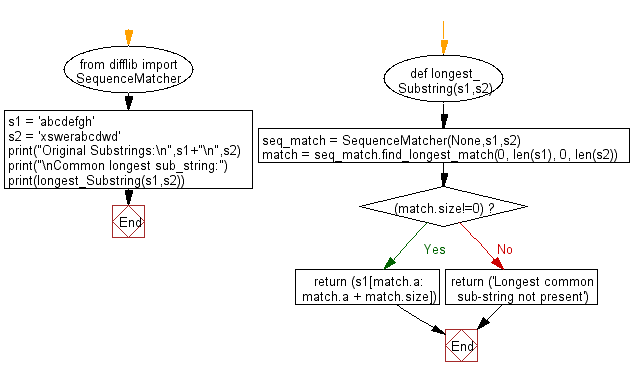﻿ Python: Find the longest common sub-string from two given strings - w3resource# Python: Find the longest common sub-string from two given strings

## Python String: Exercise-69 with Solution

Write a Python program to find the longest common sub-string from two given strings.

Sample Solution:-

Python Code:

``````from difflib import SequenceMatcher

def longest_Substring(s1,s2):

seq_match = SequenceMatcher(None,s1,s2)

match = seq_match.find_longest_match(0, len(s1), 0, len(s2))

# return the longest substring
if (match.size!=0):
return (s1[match.a: match.a + match.size])
else:
return ('Longest common sub-string not present')

s1 = 'abcdefgh'
s2 = 'xswerabcdwd'
print("Original Substrings:\n",s1+"\n",s2)
print("\nCommon longest sub_string:")
print(longest_Substring(s1,s2))
```
```

Sample Output:

```Original Substrings:
abcdefgh
xswerabcdwd

Common longest sub_string:
abcd
Original Substrings:
abcdefgh
xswerabcdwd

Common longest sub_string:
abcd
```

Pictorial Presentation:Flowchart:Python Code Editor:

Have another way to solve this solution? Contribute your code (and comments) through Disqus.

What is the difficulty level of this exercise?

Test your Programming skills with w3resource's quiz.

﻿

## Python: Tips of the Day

Check if a given key already exists in a dictionary:

In is the intended way to test for the existence of a key in a dict.

```d = {"key1": 10, "key2": 23}

if "key1" in d:
print("this will execute")

if "nonexistent key" in d:
print("this will not")
```

If you wanted a default, you can always use dict.get():

```d = dict()

for i in range(100):
key = i % 10
d[key] = d.get(key, 0) + 1
```

and if you wanted to always ensure a default value for any key you can either use dict.setdefault() repeatedly or defaultdict from the collections module, like so:

```from collections import defaultdict

d = defaultdict(int)

for i in range(100):
d[i % 10] += 1
```

but in general, the in keyword is the best way to do it.

Ref: https://bit.ly/2XPMRyz